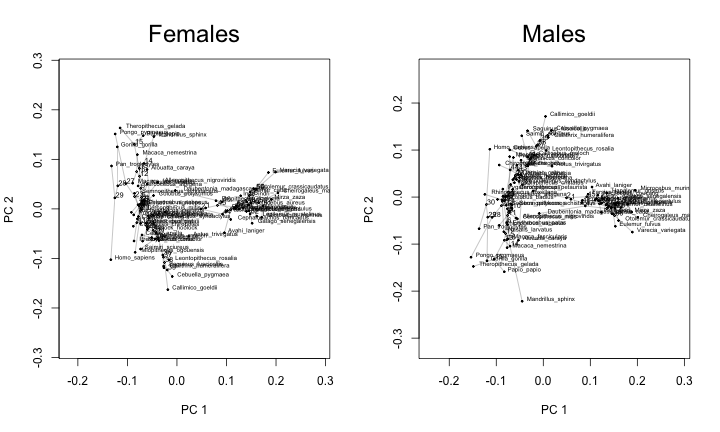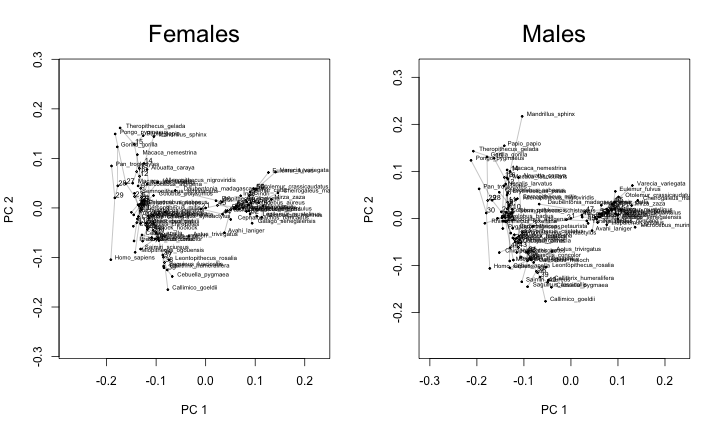Back to blog

## Flipping PC axes in geomorph plotGMPhyloMorphoSpace plots

The `geomorph` R package provides a convenient function, `plotGMPhyloMorphoSpace`, for plotting a phylogeny and Procrustes aligned specimens in tangent space (Rohlf 2002; Klingenberg & Gidaszewski 2010). However, a limitation of this function is that it does not allow users to flip the direction of one or both principal axes. Although this might be a rarely used feature, I recently found myself in a situation where I wanted to do exactly this. In this post, I reproduce my situation and make a simple modification to `plotGMPhyloMorphoSpace` that allows the user to flip one or both principal axes.

### The situation

I have geometric morphometric data on skull shape for a sample of 63-64 primate species, with one male and one female specimen for each species (with a couple of exceptions). I am analyzing males and females separately, but given the strong correlation between male and female skulls from the same species, the analyses should produce similar results. I already cleaned and aligned the two datasets with Generalized Procrustes Alignment, and obtained phylogenetic trees for each dataset. The data can be imported from my website like this:

``````# load packages
library("geomorph")

# import GPA aligned data for females and males

# import phylogenies for females and males
``````

Now, generate side-by-side phylomorphospace plots. The plot parameters here serve to make the text a bit more readable, at least for peripheral species:

``````# side-by-side phylomorphospace plots
layout(matrix(1:2, 1, 2))
plotGMPhyloMorphoSpace(tree.f, gpa.f\$coords, plot.param=list(t.cex=0.3, n.cex=0.3, lwd=0.3, txt.cex=0.5))
mtext("Females", line=1, cex=2)
plotGMPhyloMorphoSpace(tree.m, gpa.m\$coords, plot.param=list(t.cex=0.3, n.cex=0.3, lwd=0.3, txt.cex=0.5))
mtext("Males", line=1, cex=2)
``````There isn’t anything wrong with these plots, but it is annoying that the y-axes in the two plots seem to go in opposite directions even though they actually describe the same axis of variation. In other words, the second plot looks an awful lot like the first plot if it were vertically flipped. This mismatch in plot orientations makes it more difficult to recognize that the second principal axis describes a very similar gradient, and more difficult to compare differences between the plots.

Since the direction of principal axes are arbitrary, we are allowed to flip the y-axis in the first plot to match the second plot. However, since the `plotGMPhyloMorphoSpace` function doesn’t include a way to do this, I had to make a slight modification to the function. The complete code for the modified function can be found here on GitHub, and the only changes are two additional lines of code (87-88). The original function already includes `xaxis` and `yaxis` arguments that allow the user to specify which principal axes are represented on the x and y-axes, respectively. In the original function, these arguments must be positive integers, and they default to 1 and 2. With my modification, you can specify a flipped axis by providing a negative integer for these arguments. For example, to flip the y-axis in the second plot, I would simply specify `yaxis = -2`.

Here is how to load the function and produce the plots I want:

``````# import function from GitHub
source(url("https://raw.githubusercontent.com/rgriff23/Dissertation/master/Chapter_5/R/plotGMPhyloMorphoSpace_axisflip.R"))

# side-by-side phylomorphospace plots with y-axis in male plot flipped
layout(matrix(1:2, 1, 2))
plotGMPhyloMorphoSpace_axisflip(tree.f, gpa.f\$coords, plot.param=list(t.cex=0.3, n.cex=0.3, lwd=0.3, txt.cex=0.5))
mtext("Females", line=1, cex=2)
plotGMPhyloMorphoSpace_axisflip(tree.m, gpa.m\$coords, yaxis=-2, plot.param=list(t.cex=0.3, n.cex=0.3, lwd=0.3, txt.cex=0.5))
mtext("Males", line=1, cex=2)
``````Now it is much easier to see that the two plots are quite similar, and it is also easier to compare their slight differences side by side (for example, the male mandrill stands out from the pack much moreso than the female).

References

Klingenberg, C. P., and N. A. Gidaszewski. 2010. Testing and quantifying phylogenetic signals and homoplasy in morphometric data. Syst. Biol. 59:245-261.

Rohlf, F. J. 2002. Geometric morphometrics and phylogeny. Pp.175-193 in N. Macleod, and P. Forey, eds. Morphology, shape, and phylogeny. Taylor & Francis, London.

Back to blog